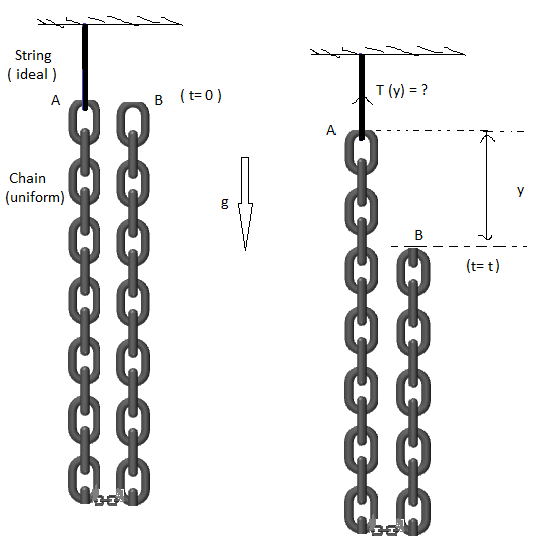# What a chain! 4A chain of uniform mass density $\lambda$ and mass $M$ and length $L$ is hanging from ceiling through a string. The other end of the chain is held at rest at the position shown in the figure. At time $t=0$ the held part of chain is left to fall under gravity. Find the tension in the string as a function of time $(t)$ (neglecting friction). Your answer can be represented as

$T(t) = \frac{Mg}{a} + \frac{b Mg^2t^2}{c L}$

where $a$, $b$, and $c$ are positive coprime integers. Enter your answer as $a + b + c$.

Note: Figures are not to scale.

This is inspired by Depanshu's Chain please don't fall! and is a part of my set Aniket's Mechanics Challenges.

×